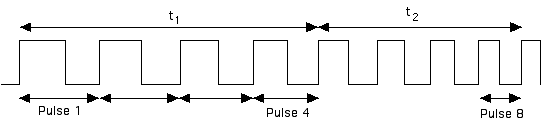# Acceleration Calculation

The Super Pulley has very free-running bearings so the rotation of the pulley matches the motion of the string, which matches the motion of the hanger and cart. The Super Pulley has ten spokes and the circumference of its groove is exactly 15 cm.

Every time a spoke cuts the light beam in the photogate a voltage pulse is created and the Smart Timer times its duration. It times the first 8 pulses in two groups of 4. We can call the duration of these time intervals t1 and t2.

During each time interval, the cart moves 4 spokes-worth of a full turn, which is 4/10 of 15 cm or 6 cm.

Its average speed during each interval is 6/ t1 and 6/ t2 cms-1.

The extra speed gained is the difference between these average speeds, 6/ t2  ­- 6/ t1.

The actual speed of the cart is usually very close to its average speed at the middle of the time interval. The time between the two average speed measurements is the time between the middle of t1, which occurs at t1 /2, and the middle of t2, which occurs at t1 + t2 /2. This time is therefore t1 + t2 /2 – t1 /2 or (t2  ­+ t1)/2.

The acceleration is the extra speed gained per second, which is

(6/ t2  ­- 6/ t1)/(t2  ­- t1)/2 or 12(1/ t2  ­- 1/ t1)/(t2  ­+ t1).

This is the calculation the Smart Timer is programmed to perform. Ticker timer measurements need a similar calculation; the only difference being that the Smart Timer measures successive times for two equal displacements whereas the Ticker Timer measures successive displacements for two equal times.